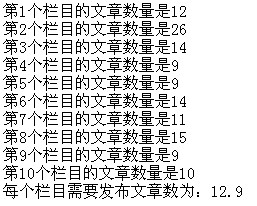# 数组介绍和实例

[日期：2010-03-16]   来源：IT部落窝  作者：IT部落窝   阅读：919[字体： ]

asp中的数组分为一维数组和多维数组。最常用的是二维数组。数组的写法为：变量名（下标）。下标是一个数值，用来说明自己是数组中的第几个变量。

实例一：数组的简单用法，做一个加法运算。
代码如下：
<%
dim a(2)   '此部分是注释：这条语句的作用是告诉asp系统，要使用一个数组，数组的变量名为a，要使用3个数组元素，数组元素是从0开始

a(0)=5
a(1)=8
a(2)=2
response.write(a(0)+a(1)+a(2))
%>

实例二：利用数组，求网站每个栏目需要平均发表多少篇文章，才能达到平均数。
代码如下：
<%
dim a(9)
a(0)=12
a(1)=26
a(2)=14
a(3)=9
a(4)=9
a(5)=14
a(6)=11
a(7)=15
a(8)=9
a(9)=10
for i=0 to 9
response.write("第"&(i+1) &"个栏目的文章数量是"&a(i)&"<br>")
b=b+a(i)
next
response.write("每个栏目需要发布文章数为："&b/10)
%>分享到： QQ空间 新浪微博 腾讯微博 人人网